# Computing a covariance matrix¶

Many methods in MNE, including source estimation and some classification algorithms, require covariance estimations from the recordings. In this tutorial we cover the basics of sensor covariance computations and construct a noise covariance matrix that can be used when computing the minimum-norm inverse solution. For more information, see The minimum-norm current estimates.

import os.path as op

import mne
from mne.datasets import sample


Source estimation method such as MNE require a noise estimations from the recordings. In this tutorial we cover the basics of noise covariance and construct a noise covariance matrix that can be used when computing the inverse solution. For more information, see The minimum-norm current estimates.

data_path = sample.data_path()
raw_empty_room_fname = op.join(
data_path, 'MEG', 'sample', 'ernoise_raw.fif')
raw_fname = op.join(data_path, 'MEG', 'sample', 'sample_audvis_raw.fif')
raw.set_eeg_reference('average', projection=True)


Out:

Opening raw data file /home/circleci/mne_data/MNE-sample-data/MEG/sample/ernoise_raw.fif...
Read a total of 3 projection items:
PCA-v1 (1 x 102)  idle
PCA-v2 (1 x 102)  idle
PCA-v3 (1 x 102)  idle
Range : 19800 ... 85867 =     32.966 ...   142.965 secs
Opening raw data file /home/circleci/mne_data/MNE-sample-data/MEG/sample/sample_audvis_raw.fif...
Read a total of 3 projection items:
PCA-v1 (1 x 102)  idle
PCA-v2 (1 x 102)  idle
PCA-v3 (1 x 102)  idle
Range : 25800 ... 192599 =     42.956 ...   320.670 secs
1 projection items deactivated


The definition of noise depends on the paradigm. In MEG it is quite common to use empty room measurements for the estimation of sensor noise. However if you are dealing with evoked responses, you might want to also consider resting state brain activity as noise. First we compute the noise using empty room recording. Note that you can also use only a part of the recording with tmin and tmax arguments. That can be useful if you use resting state as a noise baseline. Here we use the whole empty room recording to compute the noise covariance (tmax=None is the same as the end of the recording, see mne.compute_raw_covariance()).

Keep in mind that you want to match your empty room dataset to your actual MEG data, processing-wise. Ensure that filters are all the same and if you use ICA, apply it to your empty-room and subject data equivalently. In this case we did not filter the data and we don’t use ICA. However, we do have bad channels and projections in the MEG data, and, hence, we want to make sure they get stored in the covariance object.

raw_empty_room.info['bads'] = [
bb for bb in raw.info['bads'] if 'EEG' not in bb]
[pp.copy() for pp in raw.info['projs'] if 'EEG' not in pp['desc']])

noise_cov = mne.compute_raw_covariance(
raw_empty_room, tmin=0, tmax=None)


Out:

3 projection items deactivated
Using up to 550 segments
Number of samples used : 66000
[done]


Now that you have the covariance matrix in an MNE-Python object you can save it to a file with mne.write_cov(). Later you can read it back using mne.read_cov().

You can also use the pre-stimulus baseline to estimate the noise covariance. First we have to construct the epochs. When computing the covariance, you should use baseline correction when constructing the epochs. Otherwise the covariance matrix will be inaccurate. In MNE this is done by default, but just to be sure, we define it here manually.

events = mne.find_events(raw)
epochs = mne.Epochs(raw, events, event_id=1, tmin=-0.2, tmax=0.5,
baseline=(-0.2, 0.0), decim=3,  # we'll decimate for speed
verbose='error')  # and ignore the warning about aliasing


Out:

320 events found
Event IDs: [ 1  2  3  4  5 32]


Note that this method also attenuates any activity in your source estimates that resemble the baseline, if you like it or not.

noise_cov_baseline = mne.compute_covariance(epochs, tmax=0)


Out:

Computing rank from data with rank=None
Using tolerance 3.7e-09 (2.2e-16 eps * 305 dim * 5.4e+04  max singular value)
Estimated rank (mag + grad): 302
MEG: rank 302 computed from 305 data channels with 3 projectors
Using tolerance 1.8e-11 (2.2e-16 eps * 59 dim * 1.4e+03  max singular value)
Estimated rank (eeg): 58
EEG: rank 58 computed from 59 data channels with 1 projector
Created an SSP operator (subspace dimension = 4)
Setting small MEG eigenvalues to zero (without PCA)
Setting small EEG eigenvalues to zero (without PCA)
Reducing data rank from 364 -> 360
Estimating covariance using EMPIRICAL
Done.
Number of samples used : 2952
[done]


## Plot the covariance matrices¶

Try setting proj to False to see the effect. Notice that the projectors in epochs are already applied, so proj parameter has no effect.

noise_cov.plot(raw_empty_room.info, proj=True)
noise_cov_baseline.plot(epochs.info, proj=True)

•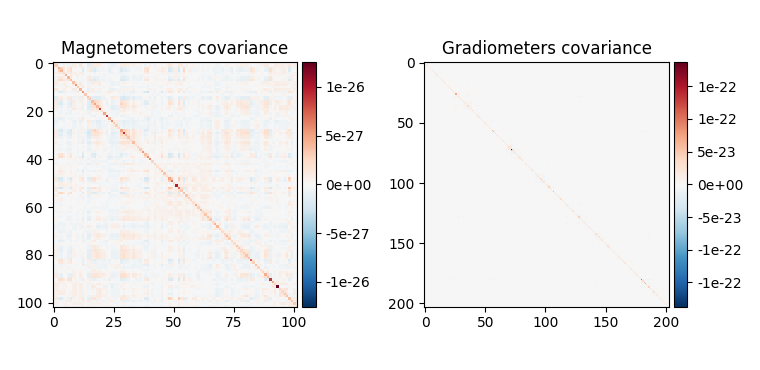•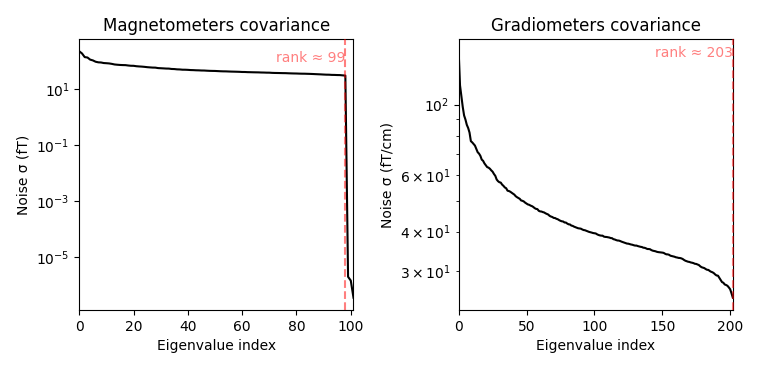•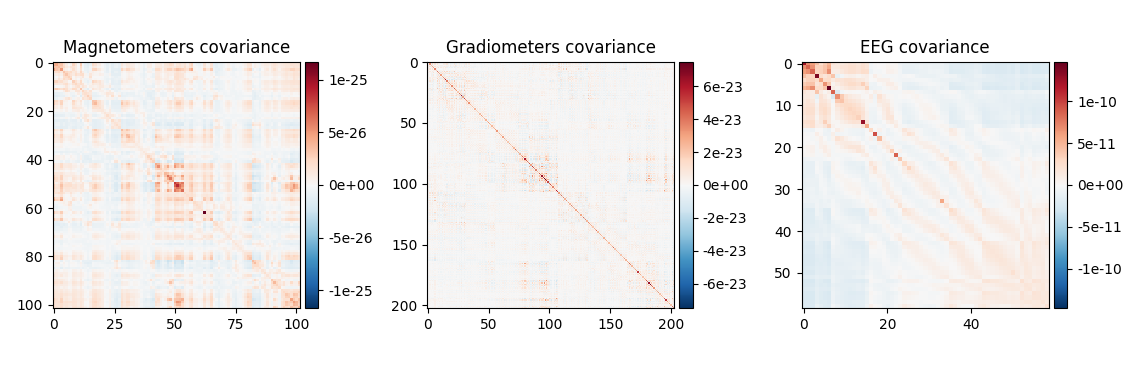•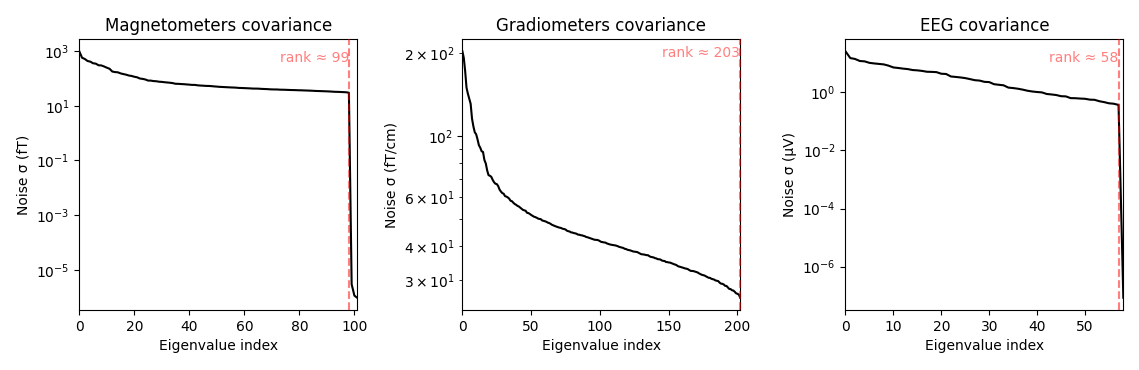Out:

    Created an SSP operator (subspace dimension = 3)
Computing rank from covariance with rank=None
Using tolerance 1.2e-15 (2.2e-16 eps * 102 dim * 0.052  max singular value)
Estimated rank (mag): 99
MAG: rank 99 computed from 102 data channels with 0 projectors
Computing rank from covariance with rank=None
Using tolerance 9.7e-14 (2.2e-16 eps * 203 dim * 2.2  max singular value)
GRAD: rank 203 computed from 203 data channels with 0 projectors
Created an SSP operator (subspace dimension = 4)
Computing rank from covariance with rank=None
Using tolerance 2.2e-14 (2.2e-16 eps * 102 dim * 0.96  max singular value)
Estimated rank (mag): 99
MAG: rank 99 computed from 102 data channels with 0 projectors
Computing rank from covariance with rank=None
Using tolerance 1.9e-13 (2.2e-16 eps * 203 dim * 4.1  max singular value)
GRAD: rank 203 computed from 203 data channels with 0 projectors
Computing rank from covariance with rank=None
Using tolerance 8.4e-14 (2.2e-16 eps * 59 dim * 6.4  max singular value)
Estimated rank (eeg): 58
EEG: rank 58 computed from 59 data channels with 0 projectors


## How should I regularize the covariance matrix?¶

The estimated covariance can be numerically unstable and tends to induce correlations between estimated source amplitudes and the number of samples available. The MNE manual therefore suggests to regularize the noise covariance matrix (see Regularization of the noise-covariance matrix), especially if only few samples are available. Unfortunately it is not easy to tell the effective number of samples, hence, to choose the appropriate regularization. In MNE-Python, regularization is done using advanced regularization methods described in 1. For this the ‘auto’ option can be used. With this option cross-validation will be used to learn the optimal regularization:

noise_cov_reg = mne.compute_covariance(epochs, tmax=0., method='auto',
rank=None)


Out:

Computing rank from data with rank=None
Using tolerance 3.7e-09 (2.2e-16 eps * 305 dim * 5.4e+04  max singular value)
Estimated rank (mag + grad): 302
MEG: rank 302 computed from 305 data channels with 3 projectors
Using tolerance 1.8e-11 (2.2e-16 eps * 59 dim * 1.4e+03  max singular value)
Estimated rank (eeg): 58
EEG: rank 58 computed from 59 data channels with 1 projector
Created an SSP operator (subspace dimension = 4)
Setting small MEG eigenvalues to zero (without PCA)
Setting small EEG eigenvalues to zero (without PCA)
Reducing data rank from 364 -> 360
Estimating covariance using SHRUNK
Done.
Estimating covariance using DIAGONAL_FIXED
MAG regularization : 0.1
EEG regularization : 0.1
Done.
Estimating covariance using EMPIRICAL
Done.
Using cross-validation to select the best estimator.
MAG regularization : 0.1
EEG regularization : 0.1
MAG regularization : 0.1
EEG regularization : 0.1
MAG regularization : 0.1
EEG regularization : 0.1
Number of samples used : 2952
log-likelihood on unseen data (descending order):
shrunk: -1768.885
diagonal_fixed: -1785.429
empirical: -1797.005
selecting best estimator: shrunk
[done]


This procedure evaluates the noise covariance quantitatively by how well it whitens the data using the negative log-likelihood of unseen data. The final result can also be visually inspected. Under the assumption that the baseline does not contain a systematic signal (time-locked to the event of interest), the whitened baseline signal should be follow a multivariate Gaussian distribution, i.e., whitened baseline signals should be between -1.96 and 1.96 at a given time sample. Based on the same reasoning, the expected value for the global field power (GFP) is 1 (calculation of the GFP should take into account the true degrees of freedom, e.g. ddof=3 with 2 active SSP vectors):

evoked = epochs.average()
evoked.plot_white(noise_cov_reg, time_unit='s')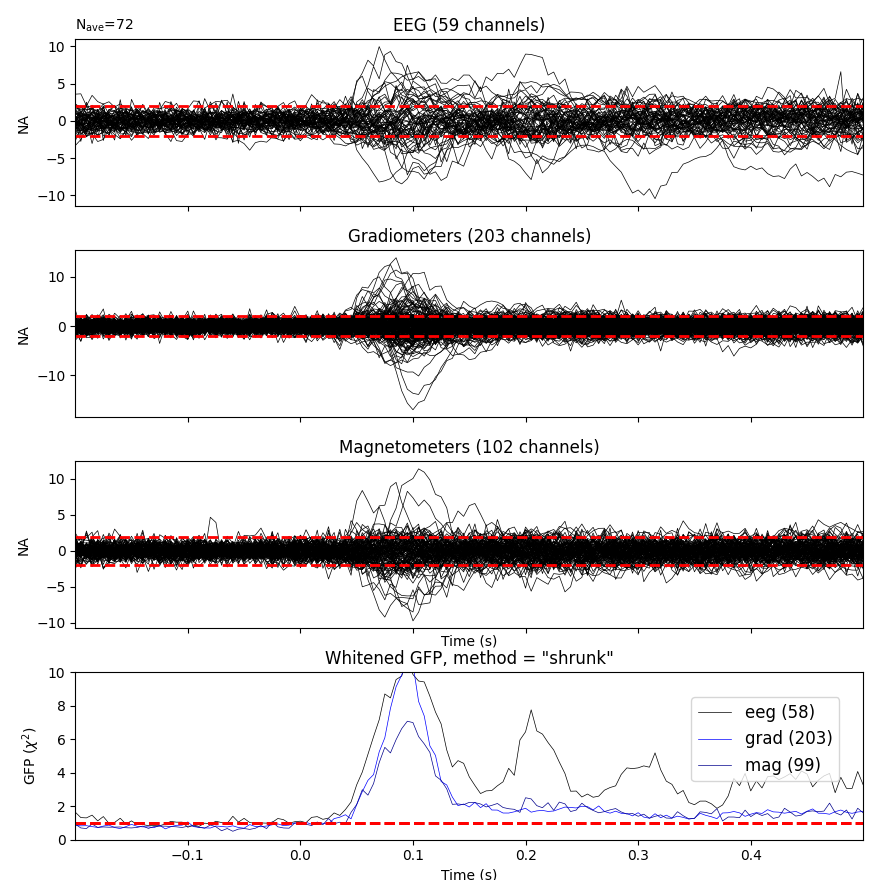Out:

Computing rank from covariance with rank=None
Using tolerance 8.4e-14 (2.2e-16 eps * 59 dim * 6.4  max singular value)
Estimated rank (eeg): 58
EEG: rank 58 computed from 59 data channels with 1 projector
Computing rank from covariance with rank=None
Using tolerance 1.8e-13 (2.2e-16 eps * 203 dim * 4  max singular value)
GRAD: rank 203 computed from 203 data channels with 0 projectors
Computing rank from covariance with rank=None
Using tolerance 2.1e-14 (2.2e-16 eps * 102 dim * 0.91  max singular value)
Estimated rank (mag): 99
MAG: rank 99 computed from 102 data channels with 3 projectors
Created an SSP operator (subspace dimension = 4)
Computing rank from covariance with rank={'eeg': 58, 'grad': 203, 'mag': 99, 'meg': 302}
Setting small MEG eigenvalues to zero (without PCA)
Setting small EEG eigenvalues to zero (without PCA)
Created the whitener using a noise covariance matrix with rank 360 (4 small eigenvalues omitted)


This plot displays both, the whitened evoked signals for each channels and the whitened GFP. The numbers in the GFP panel represent the estimated rank of the data, which amounts to the effective degrees of freedom by which the squared sum across sensors is divided when computing the whitened GFP. The whitened GFP also helps detecting spurious late evoked components which can be the consequence of over- or under-regularization.

Note that if data have been processed using signal space separation (SSS) 2, gradiometers and magnetometers will be displayed jointly because both are reconstructed from the same SSS basis vectors with the same numerical rank. This also implies that both sensor types are not any longer statistically independent. These methods for evaluation can be used to assess model violations. Additional introductory materials can be found here.

For expert use cases or debugging the alternative estimators can also be compared (see Whitening evoked data with a noise covariance) and Demonstrate impact of whitening on source estimates):

noise_covs = mne.compute_covariance(
epochs, tmax=0., method=('empirical', 'shrunk'), return_estimators=True,
rank=None)
evoked.plot_white(noise_covs, time_unit='s')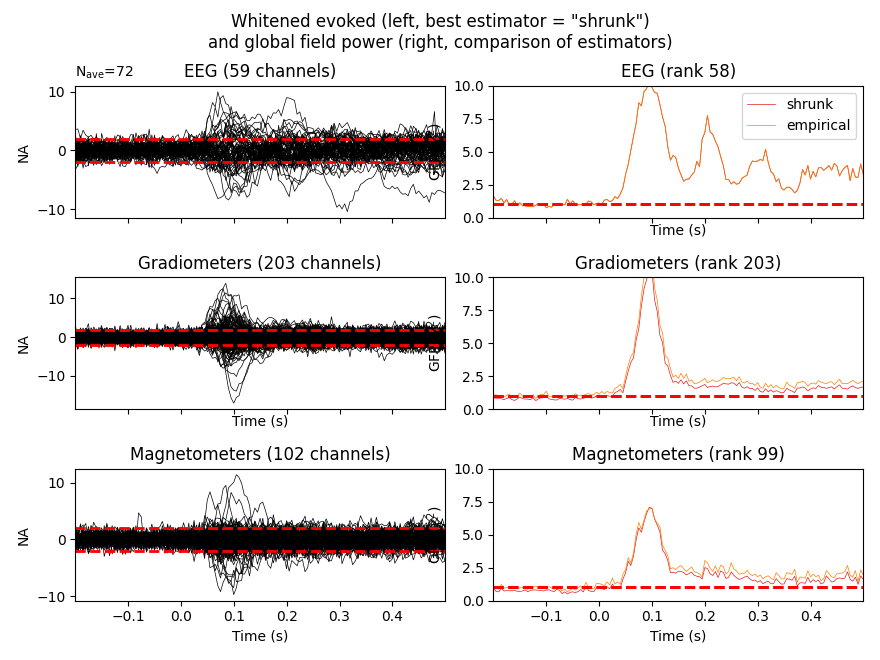Out:

Computing rank from data with rank=None
Using tolerance 3.7e-09 (2.2e-16 eps * 305 dim * 5.4e+04  max singular value)
Estimated rank (mag + grad): 302
MEG: rank 302 computed from 305 data channels with 3 projectors
Using tolerance 1.8e-11 (2.2e-16 eps * 59 dim * 1.4e+03  max singular value)
Estimated rank (eeg): 58
EEG: rank 58 computed from 59 data channels with 1 projector
Created an SSP operator (subspace dimension = 4)
Setting small MEG eigenvalues to zero (without PCA)
Setting small EEG eigenvalues to zero (without PCA)
Reducing data rank from 364 -> 360
Estimating covariance using EMPIRICAL
Done.
Estimating covariance using SHRUNK
Done.
Using cross-validation to select the best estimator.
Number of samples used : 2952
log-likelihood on unseen data (descending order):
shrunk: -1768.885
empirical: -1797.005
[done]
Computing rank from covariance with rank=None
Using tolerance 8.4e-14 (2.2e-16 eps * 59 dim * 6.4  max singular value)
Estimated rank (eeg): 58
EEG: rank 58 computed from 59 data channels with 1 projector
Computing rank from covariance with rank=None
Using tolerance 1.8e-13 (2.2e-16 eps * 203 dim * 4  max singular value)
GRAD: rank 203 computed from 203 data channels with 0 projectors
Computing rank from covariance with rank=None
Using tolerance 2.1e-14 (2.2e-16 eps * 102 dim * 0.91  max singular value)
Estimated rank (mag): 99
MAG: rank 99 computed from 102 data channels with 3 projectors
Computing rank from covariance with rank=None
Using tolerance 8.4e-14 (2.2e-16 eps * 59 dim * 6.4  max singular value)
Estimated rank (eeg): 58
EEG: rank 58 computed from 59 data channels with 1 projector
Computing rank from covariance with rank=None
Using tolerance 1.9e-13 (2.2e-16 eps * 203 dim * 4.1  max singular value)
GRAD: rank 203 computed from 203 data channels with 0 projectors
Computing rank from covariance with rank=None
Using tolerance 2.2e-14 (2.2e-16 eps * 102 dim * 0.96  max singular value)
Estimated rank (mag): 99
MAG: rank 99 computed from 102 data channels with 3 projectors
Created an SSP operator (subspace dimension = 4)
Computing rank from covariance with rank={'eeg': 58, 'grad': 203, 'mag': 99, 'meg': 302}
Setting small MEG eigenvalues to zero (without PCA)
Setting small EEG eigenvalues to zero (without PCA)
Created the whitener using a noise covariance matrix with rank 360 (4 small eigenvalues omitted)
Created an SSP operator (subspace dimension = 4)
Computing rank from covariance with rank={'eeg': 58, 'grad': 203, 'mag': 99, 'meg': 302}
Setting small MEG eigenvalues to zero (without PCA)
Setting small EEG eigenvalues to zero (without PCA)
Created the whitener using a noise covariance matrix with rank 360 (4 small eigenvalues omitted)


This will plot the whitened evoked for the optimal estimator and display the GFPs for all estimators as separate lines in the related panel.

Finally, let’s have a look at the difference between empty room and event related covariance, hacking the “method” option so that their types are shown in the legend of the plot.

evoked_meg = evoked.copy().pick('meg')
noise_cov['method'] = 'empty_room'
noise_cov_baseline['method'] = 'baseline'
evoked_meg.plot_white([noise_cov_baseline, noise_cov], time_unit='s')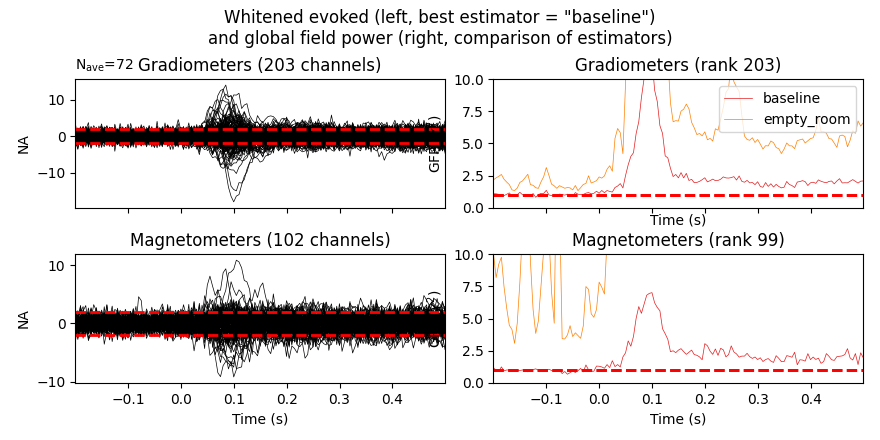Out:

Removing projector <Projection | Average EEG reference, active : True, n_channels : 59>
Computing rank from covariance with rank=None
Using tolerance 1.9e-13 (2.2e-16 eps * 203 dim * 4.1  max singular value)
GRAD: rank 203 computed from 203 data channels with 0 projectors
Computing rank from covariance with rank=None
Using tolerance 2.2e-14 (2.2e-16 eps * 102 dim * 0.96  max singular value)
Estimated rank (mag): 99
MAG: rank 99 computed from 102 data channels with 3 projectors
Computing rank from covariance with rank=None
Using tolerance 9.7e-14 (2.2e-16 eps * 203 dim * 2.2  max singular value)
GRAD: rank 203 computed from 203 data channels with 0 projectors
Computing rank from covariance with rank=None
Using tolerance 1.2e-15 (2.2e-16 eps * 102 dim * 0.052  max singular value)
Estimated rank (mag): 99
MAG: rank 99 computed from 102 data channels with 3 projectors
Created an SSP operator (subspace dimension = 3)
Computing rank from covariance with rank={'grad': 203, 'mag': 99, 'meg': 302}
Setting small MEG eigenvalues to zero (without PCA)
Created the whitener using a noise covariance matrix with rank 302 (3 small eigenvalues omitted)
Created an SSP operator (subspace dimension = 3)
Computing rank from covariance with rank={'grad': 203, 'mag': 99, 'meg': 302}
Setting small MEG eigenvalues to zero (without PCA)
Created the whitener using a noise covariance matrix with rank 302 (3 small eigenvalues omitted)


Based on the negative log-likelihood, the baseline covariance seems more appropriate. See Demonstrate impact of whitening on source estimates for more information.